in 技术 with 3,019 views and 1 comment

ABSTRACT：This paper mainly discusses how to construct a kind of intelligent learning system under the network learning space from five aspects. It not only constructs the design from the whole, but also carries on the scientific system to the data storage, the learner modeling and the knowledge analysis and code implementation. In the final push algorithm analysis, the above modules are summarized, which derived Dijkstra, UserCF, ItemCF, CAS and other algorithms, the implementation of various algorithms, and the use of Top-N performance evaluation, re-optimization, which to achieve a high-performance, high applicability of the push algorithm. The adaptive intelligence learning system, which is based on the algorithm, is a complex educational ecosystem with intelligent and flexible, balanced and harmonious and sustainable development. Only one such algorithm can be regarded as a real learning community.

KEY WORDS：intelligent learning system, recommendation algorithm, learner modeling, knowledge network

## 1. 前言

### 1.1 背景分析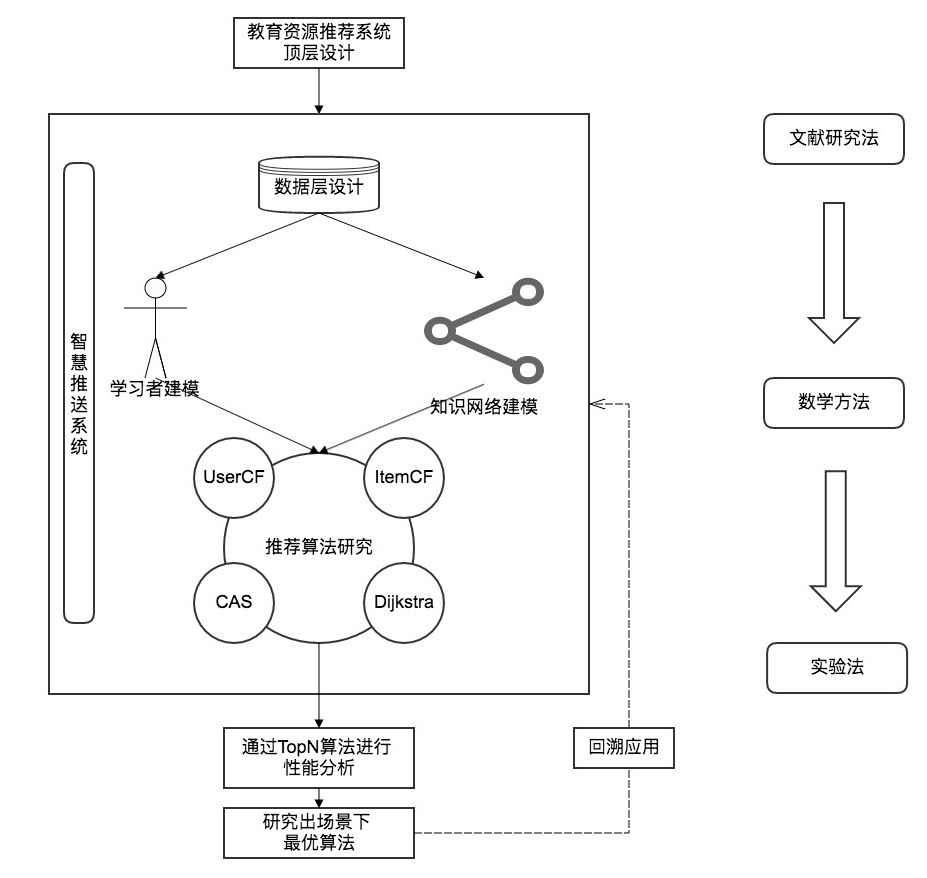## 2. 智慧学习系统顶层设计

### 2.1 智慧学习系统概述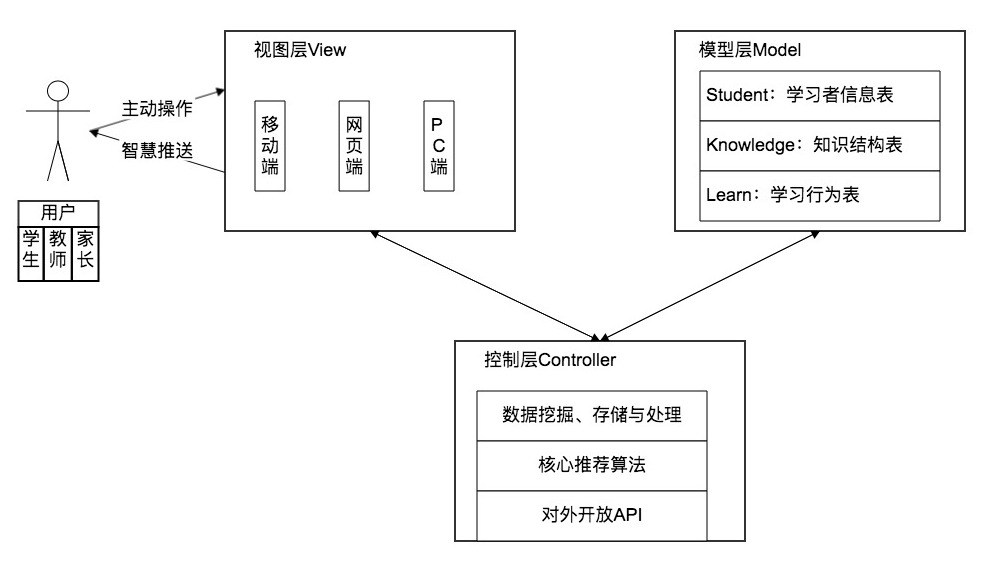### 2.2 智慧推送流程概述

st=>start: 起点
e=>end: 终点
op1=>operation: 使用系统进行学习
op2=>operation: 系统采集数据并分析
sub1=>subroutine: 扩大采集范围
cond=>condition: 是否满足算法

op3=>operation: 推荐下一个知识点

st->op1->op2->cond
cond(yes)->op3->op1
cond(no)->sub1(right)->op2

### 2.3 数据层设计

#### 2.3.1 数据层总体设计#### 2.3.2 数据挖掘层与采集层的方案设计#### 2.3.3 数据存储层设计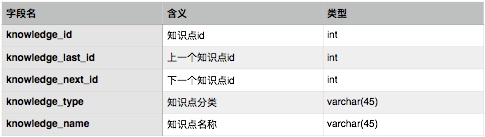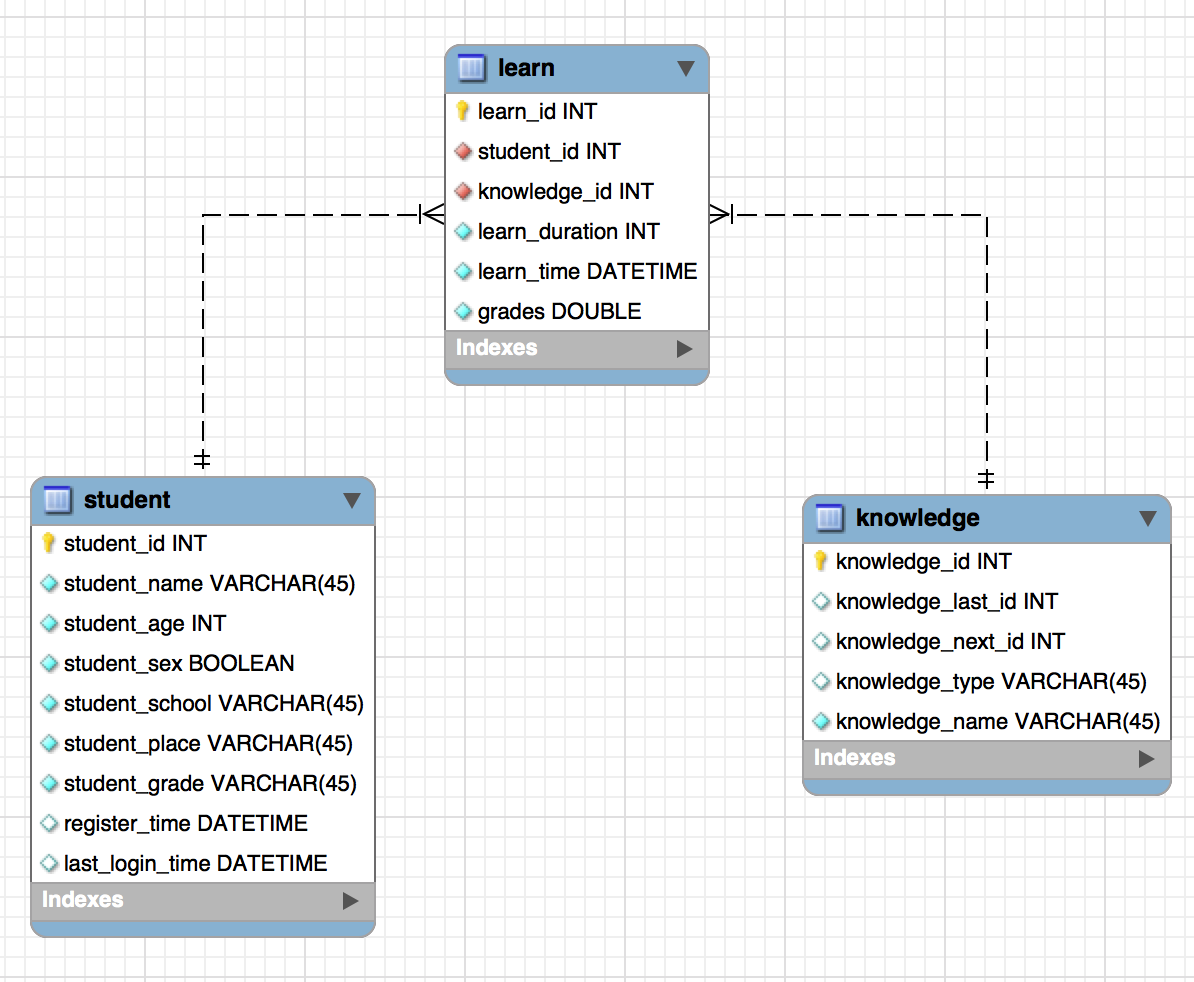#### 2.3.4 数据流处理方案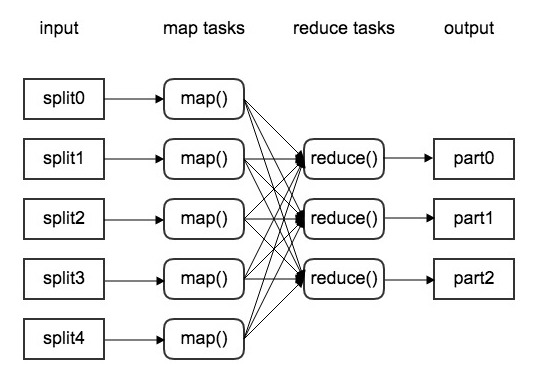## 3. 学习者特征分析与建模研究

### 3.1 学习者特征分析与建模概述

st=>start: 起点
e=>end: 终点
op1=>operation: 学习者聚类分析
op2=>operation: 学习者特征构建与选择
op3=>operation: 学习者建模与优化

st->op1->op2->op3->e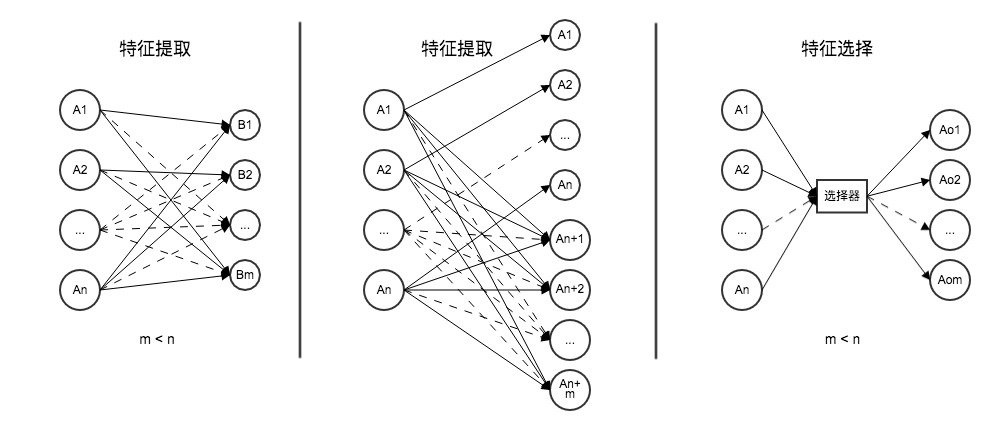### 3.2 学习者聚类分析研究

op1=>operation: 每样本单独成类
op2=>operation: 计算类间距离矩阵
op3=>operation: 合并距离最近的两类为新类
cond=>condition: 类的个数是否为1
op4=>operation: 画出聚类图
op5=>operation: 确定聚类数目和类别

op1->op2->op3->cond
cond(yes)->op4->op5
cond(no)->op2$G_1^{(0)}=\{x_1\},G_2^{(0)}=\{x_2\},G_3^{(0)}=\{x_3\}$ $G_4^{(0)}=\{x_4\},G_5^{(0)}=\{x_5\},G_6^{(0)}=\{x_6\}$

$$D^{(0)}= \begin{bmatrix} 0 & 9.540 & 8.660 & 4.900 & 4.690 & 6.780 \\ 9.540 & 0 & 10.30 & 11.79 & 10.82 & 7.140 \\ 8.660 & 10.30 & 0 & 11.09 & 9.330 & 10.15 \\ 4.900 & 11.79 & 11.09 & 0 & 6.480 & 5.830 \\ 4.690 & 10.82 & 9.330 & 6.480 & 0 & 8.120 \\ 6.780 & 7.140 & 10.15 & 5.830 & 8.120 & 0 \\ \end{bmatrix}$$

$$D^{(1)}= \begin{bmatrix} 0 & 9.540 & 8.660 & 4.900 & 6.780 \\ 9.540 & 0 & 10.30 & 11.79 & 7.140 \\ 8.660 & 10.30 & 0 & 11.09 & 10.15 \\ 4.900 & 11.79 & 11.09 & 0 & 5.830 \\ 6.780 & 7.140 & 10.15 & 5.830 & 0\\ \end{bmatrix}$$

$G_1^{(2)}=\{x_1,x_4,x_5\},G_2^{(2)}=\{x_2\}$ $G_3^{(2)}=\{x_3\},G_4^{(2)}=\{x_4\}$

$$D^{(2)}= \begin{bmatrix} 0 & 9.540 & 8.660 & 5.830 \\ 9.540 & 0 & 10.30 & 7.140 \\ 8.660 & 10.30 & 0 & 10.15 \\ 5.830 & 7.140 & 10.15 & 0 \\ \end{bmatrix}$$

$G_1^{(3)}=\{x_1,x_4,x_5,x_6\},G_2^{(3)}=\{x_2\},G_3^{(3)}=\{x_3\}$

$$D^{(3)}= \begin{bmatrix} 0 & 7.140 & 8.660 \\ 7.140 & 0 & 10.30 \\ 8.660 & 10.30 & 0 \\ \end{bmatrix}$$

$G_1^{(4)}=\{x_1,x_2,x_4,x_5,x_6\},G_2^{(4)}=\{x_3\}$

### 3.3 学习者特征构建技术与特征选择方法研究

#### 3.3.1 特征构建·

#### 3.3.2 特征选择

Gini 系数是衡量不平等性的指标，在分类问题中，分类数节点 A 的 Gini 系数表示样本在子集中被错分的可能性大小，它通常记作这个样本被选中的概率$p_i$乘以它被错分的概率$(1-p_i)$。例如响应变量 y 的取值有 k 个分类，令$p_i$是样本属于 i 类别的概率，则 Gini 系数的计算公式如下：

$Gini(A) = \sum_{i=1}^k p_i(1-p_i) = 1 - \sum_{i=1}^k p_i^2$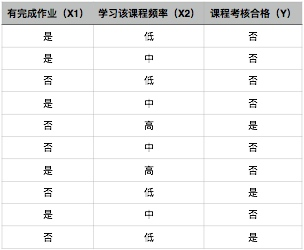$Gini(X1 = 否) = 1 - ({2\over2+3})^2 - ({3\over2+3})^2 = 0.48$

$Gini(X1 = 是) = 1 - ({5\over5+0})^2 - ({5\over5+0})^2 = 0$

$Gini(X1) = {2+3\over2+3+5+0} · Gini(X1 = 否) + {5+0\over2+3+5+0} · Gini(X1 = 是) = 0.24$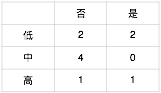$Gini(X2 = 低) = 1 - ({2\over2+2})^2 - ({2\over2+2})^2 = 0.5$

$Gini(X2 = 中) = 1 - ({4\over4+0})^2 - ({0\over4+0})^2 = 0$

$Gini(X2 = 高) = 1 - ({1\over1+1})^2 - ({1\over1+1})^2 = 0.5$

$Gini(X2) = {4\over10} · Gini(X2 = 低) + {4\over10} · Gini(X2 = 中) + {2\over10} · Gini(X2 = 高) = 0.3$

### 3.4 学习者用户画像及其建模研究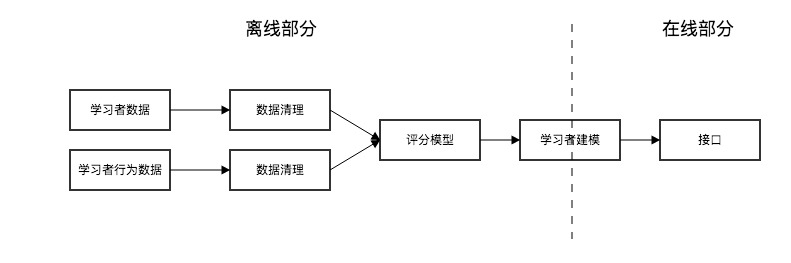·

### 3.5 模型参数优化方案研究

#### 3.5.2 使用遗传算法优化学习者模型

op1=>operation: 产生初始种群
op2=>operation: 计算适应度
cond=>condition: 是否满足

op3=>operation: 选择
op4=>operation: 交叉
op5=>operation: 变异
op6=>operation: 最佳个体

op1->op2->cond
cond(yes)->op6
cond(no)->op3(right)->op4(right)->op5(right)->op2

#### 3.5.3 使用粒子群优化算法优化学习者模型

op1=>operation: 产生初始粒子群
op2=>operation: 计算每个粒子适应度
op3=>operation: 更新pbest、gbest

cond=>condition: 是否达到最大迭代次数，

op4=>operation: 算法终止

op1->op2->op3->cond
cond(yes)->op4
cond(no)->op2

## 4. 知识网络建模研究

### 4.2 知识网络模型探究

#### 4.2.1 WS 小世界网模型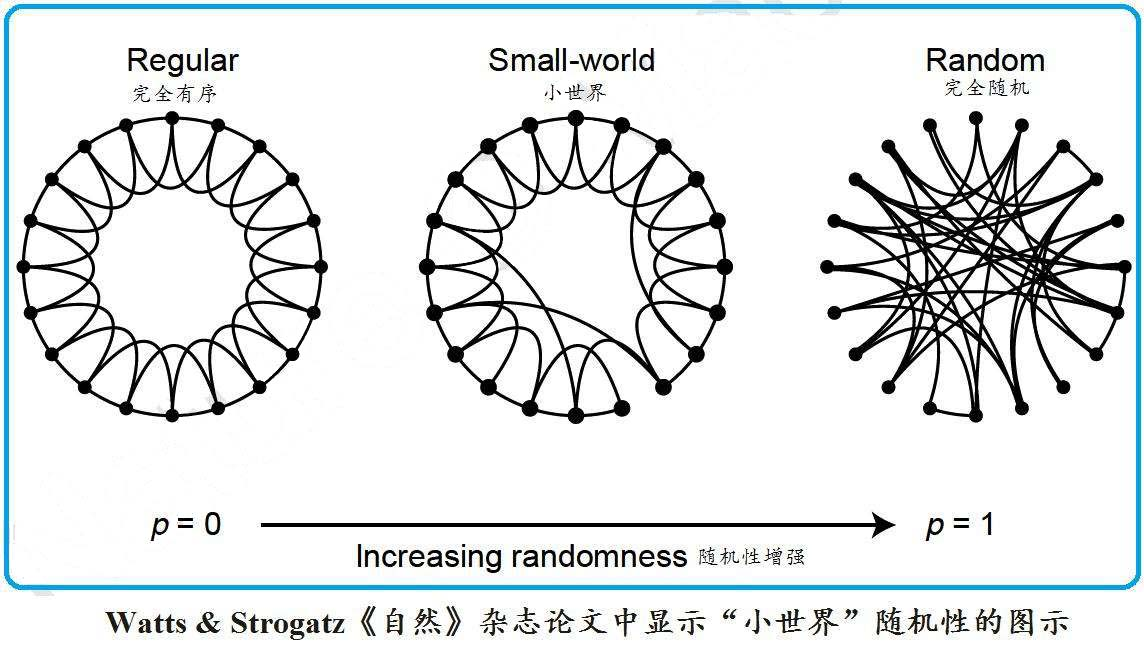#### 4.2.2 BA 无标度网模型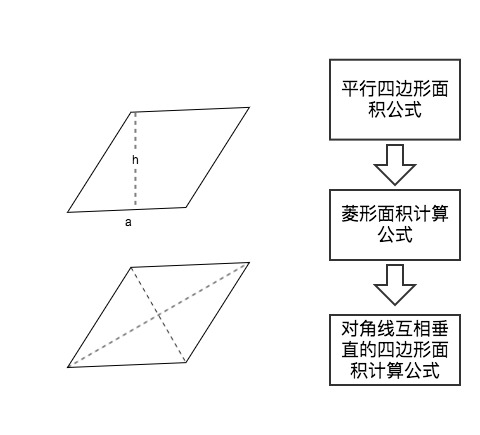### 4.3 知识网络关联分析研究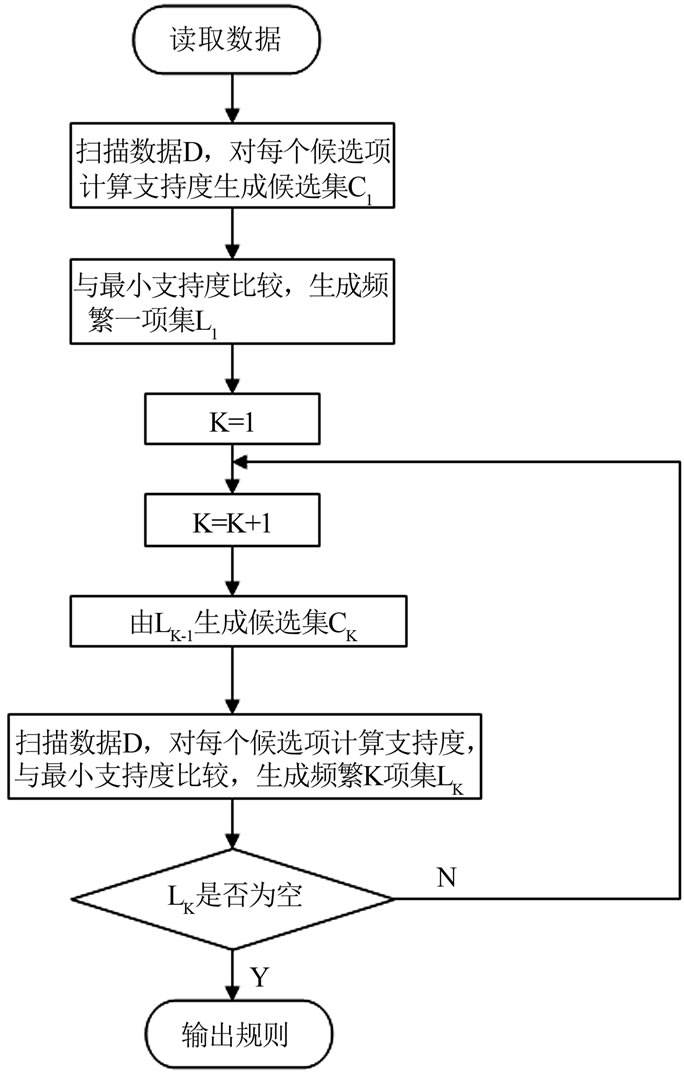1 A,C,D
2 B,C,E
3 A,B,C,E
4 B,E

{A} 2
{B} 3
{C} 3
{D} 1
{E} 3

{A} 2
{B} 3
{C} 3
{E} 3

{A,B} 1
{A,C} 2
{A,E} 1
{B,C} 2
{B,E} 3
{C,E} 2

{A,C} 2
{B,C} 2
{B,E} 3
{C,E} 2

{A,B,C} 1
{A,C,E} 1
{B,C,E} 2

{B,C,E} 2

$c(A \Rightarrow B) = {{\sigma(A \Rightarrow B)} \over || T \in D | A \subseteq T ||} = {{|| T \in D | A \cup B \subseteq T ||} \over ||T \in D | A \subseteq T||}$

$B \Rightarrow CE$ 0.67
$C \Rightarrow BE$ 0.67
$E \Rightarrow BC$ 0.67
$BC \Rightarrow E$ 1.00
$BE \Rightarrow C$ 0.67
$CE \Rightarrow B$ 1.00

Responses
1.Anton

沃德天，好6啊！膜一个～